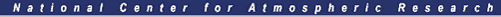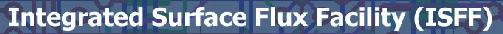# AHATS Profile Tower and Sonic Array Plots

Click on
• "plots" to see 48 hour plots centered on local noon of the given day
Most quantities are 5-minute statistics, except where noted.

Wind directions on the horizontal arrays are relative to the direction that the sonics were pointing: dir = atan(-v,u), where u and v are in instrument coordinates: +u is wind into the sonic array from the unobstructed direction. u and v have been corrected for the variability of sonic pointing angles. There is also a plot of true wind direction at the second level of the profile tower.

The nominal pointing direction of the sonics was 325 degrees with respect to true north, so: dir(true) - 325 = dir(relative)

1/2 hour power spectra of the pressure data, when available, are plotted at local noon and midnight.

Sun Mon Tues Wed Thur Fri Sat
Jun 25
plots
Jun 26
plots
Jun 27
plots
Jun 28
plots
Jun 29
plots
Jun 30
plots
Jul 01
plots
Jul 02
plots
Jul 03
plots
Jul 04
plots
Jul 05
plots
Jul 06
plots
Jul 07
plots
Jul 08
plots
Jul 09
plots
Jul 10
plots
Jul 11
plots
Jul 12
plots
Jul 13
plots
Jul 14
plots
Jul 15
plots
Jul 16
plots
Jul 17
plots
Jul 18
plots
Jul 19
plots
Jul 20
plots
Jul 21
plots
Jul 22
plots
Jul 23
plots
Jul 24
plots
Jul 25
plots
Jul 26
plots
Jul 27
plots
Jul 28
plots
Jul 29
plots
Jul 30
plots
Jul 31
plots
Aug 01
plots
Aug 02
plots
Aug 03
plots
Aug 04
plots
Aug 05
plots
Aug 06
plots
Aug 07
plots
Aug 08
plots
Aug 09
plots
Aug 10
plots
Aug 11
plots
Aug 12
plots
Aug 13
plots
Aug 14
plots
Aug 15
plots